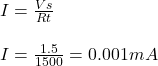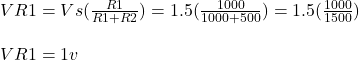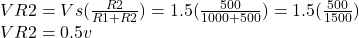Question

A 1.5 V battery is connected to a 1000 ohm resistor and a 500 ohm resistor in series. The voltage across the 1000 ohm resistor is _____ V.

1.diemthu

## 1 volt and 0.5 volt

Explanation:

Given data

voltage supplied Vs= 1.5 volts

resistance R1= 1000 ohms

resistance R2= 500 ohms

The total resistance is

Rt= 1000+ 500

Rt= 1500 ohms

The current I is given asVoltage across R1Voltage across R2In series connection the current is the same for all components while the voltage divides across all components,the voltages consumed by each individual resistance is equal to the source voltage.